# matplotlib.pyplot.subplots¶

matplotlib.pyplot.subplots(nrows=1, ncols=1, *, sharex=False, sharey=False, squeeze=True, subplot_kw=None, gridspec_kw=None, **fig_kw)[source]

Create a figure and a set of subplots.

This utility wrapper makes it convenient to create common layouts of subplots, including the enclosing figure object, in a single call.

Parameters
nrows, ncolsint, default: 1

Number of rows/columns of the subplot grid.

sharex, shareybool or {'none', 'all', 'row', 'col'}, default: False

Controls sharing of properties among x (sharex) or y (sharey) axes:

• True or 'all': x- or y-axis will be shared among all subplots.

• False or 'none': each subplot x- or y-axis will be independent.

• 'row': each subplot row will share an x- or y-axis.

• 'col': each subplot column will share an x- or y-axis.

When subplots have a shared x-axis along a column, only the x tick labels of the bottom subplot are created. Similarly, when subplots have a shared y-axis along a row, only the y tick labels of the first column subplot are created. To later turn other subplots' ticklabels on, use `tick_params`.

When subplots have a shared axis that has units, calling `set_units` will update each axis with the new units.

squeezebool, default: True
• If True, extra dimensions are squeezed out from the returned array of `Axes`:

• if only one subplot is constructed (nrows=ncols=1), the resulting single Axes object is returned as a scalar.

• for Nx1 or 1xM subplots, the returned object is a 1D numpy object array of Axes objects.

• for NxM, subplots with N>1 and M>1 are returned as a 2D array.

• If False, no squeezing at all is done: the returned Axes object is always a 2D array containing Axes instances, even if it ends up being 1x1.

subplot_kwdict, optional

Dict with keywords passed to the `add_subplot` call used to create each subplot.

gridspec_kwdict, optional

Dict with keywords passed to the `GridSpec` constructor used to create the grid the subplots are placed on.

**fig_kw

All additional keyword arguments are passed to the `pyplot.figure` call.

Returns
fig`Figure`
ax`axes.Axes` or array of Axes

ax can be either a single `Axes` object or an array of Axes objects if more than one subplot was created. The dimensions of the resulting array can be controlled with the squeeze keyword, see above.

Typical idioms for handling the return value are:

```# using the variable ax for single a Axes
fig, ax = plt.subplots()

# using the variable axs for multiple Axes
fig, axs = plt.subplots(2, 2)

# using tuple unpacking for multiple Axes
fig, (ax1, ax2) = plt.subplots(1, 2)
fig, ((ax1, ax2), (ax3, ax4)) = plt.subplots(2, 2)
```

The names `ax` and pluralized `axs` are preferred over `axes` because for the latter it's not clear if it refers to a single `Axes` instance or a collection of these.

Examples

```# First create some toy data:
x = np.linspace(0, 2*np.pi, 400)
y = np.sin(x**2)

# Create just a figure and only one subplot
fig, ax = plt.subplots()
ax.plot(x, y)
ax.set_title('Simple plot')

# Create two subplots and unpack the output array immediately
f, (ax1, ax2) = plt.subplots(1, 2, sharey=True)
ax1.plot(x, y)
ax1.set_title('Sharing Y axis')
ax2.scatter(x, y)

# Create four polar axes and access them through the returned array
fig, axs = plt.subplots(2, 2, subplot_kw=dict(projection="polar"))
axs[0, 0].plot(x, y)
axs[1, 1].scatter(x, y)

# Share a X axis with each column of subplots
plt.subplots(2, 2, sharex='col')

# Share a Y axis with each row of subplots
plt.subplots(2, 2, sharey='row')

# Share both X and Y axes with all subplots
plt.subplots(2, 2, sharex='all', sharey='all')

# Note that this is the same as
plt.subplots(2, 2, sharex=True, sharey=True)

# Create figure number 10 with a single subplot
# and clears it if it already exists.
fig, ax = plt.subplots(num=10, clear=True)
```

## Examples using `matplotlib.pyplot.subplots`¶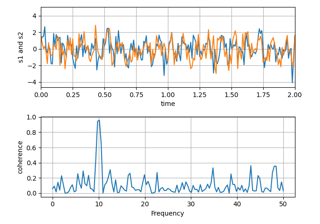Plotting the coherence of two signals¶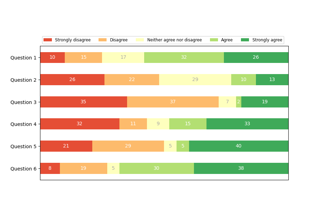Discrete distribution as horizontal bar chart¶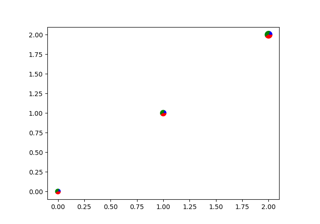Scatter plot with pie chart markers¶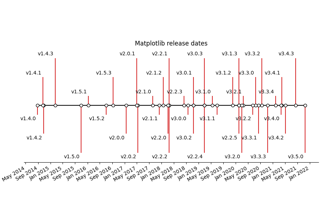Creating a timeline with lines, dates, and text¶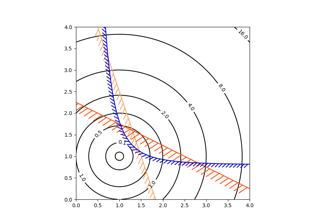Contouring the solution space of optimizations¶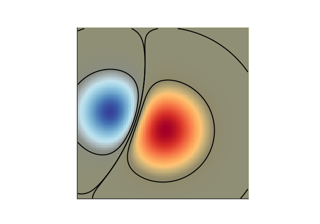Blend transparency with color in 2D images¶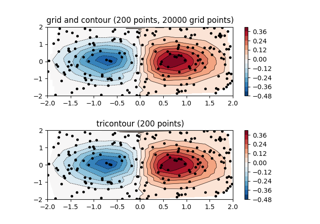Contour plot of irregularly spaced data¶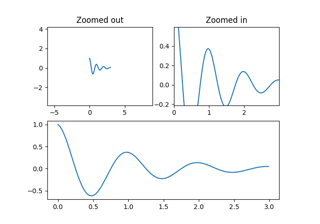Controlling view limits using margins and sticky_edges¶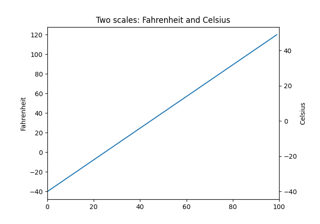Different scales on the same axes¶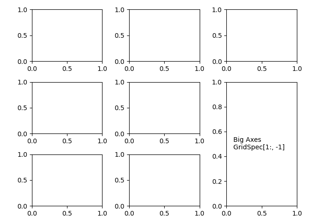Combining two subplots using subplots and GridSpec¶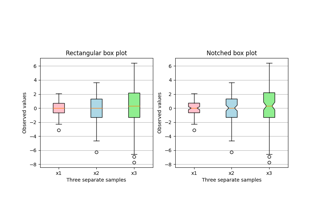Box plots with custom fill colors¶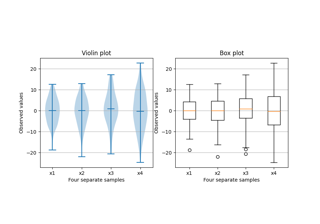Box plot vs. violin plot comparison¶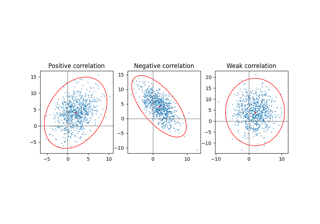Plot a confidence ellipse of a two-dimensional dataset¶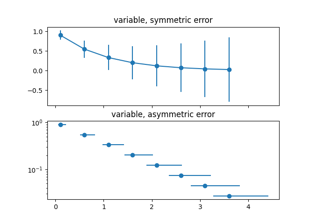Different ways of specifying error bars¶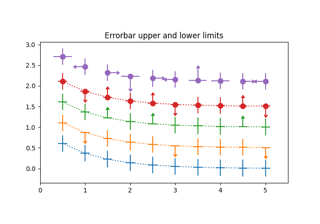Including upper and lower limits in error bars¶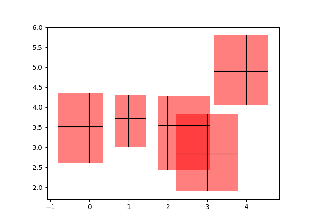Creating boxes from error bars using PatchCollection¶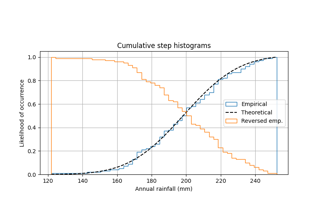Using histograms to plot a cumulative distribution¶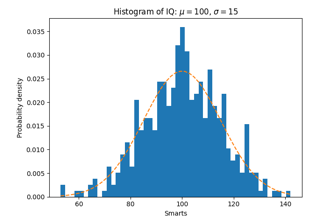Some features of the histogram (hist) function¶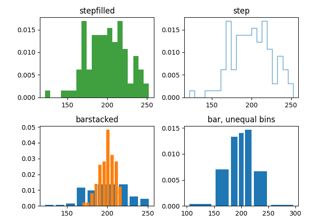Demo of the histogram function's different histtype settings¶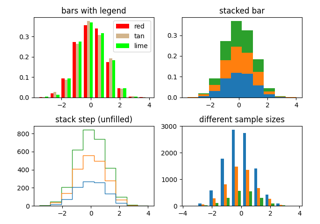The histogram (hist) function with multiple data sets¶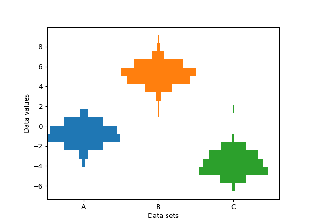Producing multiple histograms side by side¶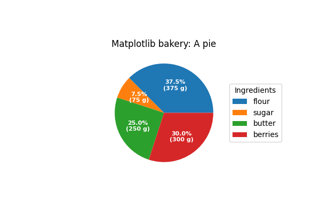Labeling a pie and a donut¶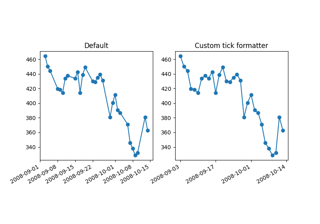Custom tick formatter for time series¶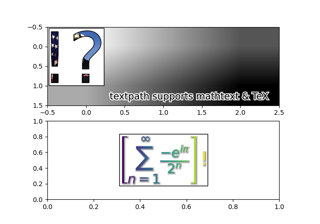Using a text as a Path¶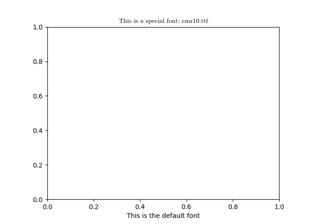Using a ttf font file in Matplotlib¶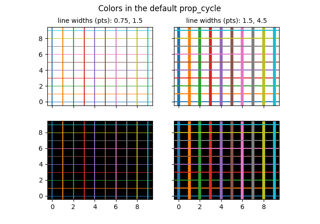Colors in the default property cycle¶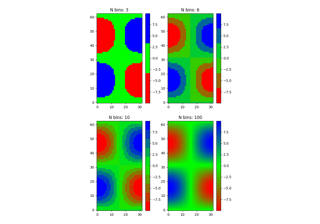Creating a colormap from a list of colors¶Line, Poly and RegularPoly Collection with autoscaling¶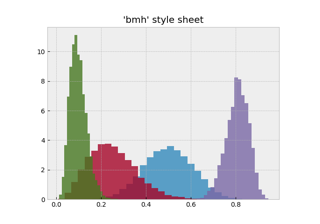Bayesian Methods for Hackers style sheet¶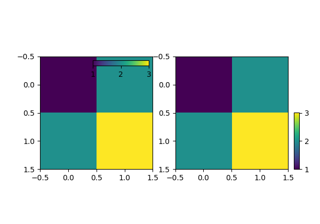Controlling the position and size of colorbars with Inset Axes¶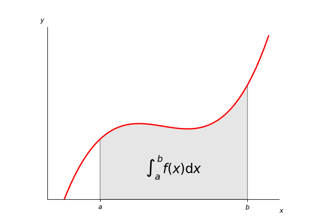Integral as the area under a curve¶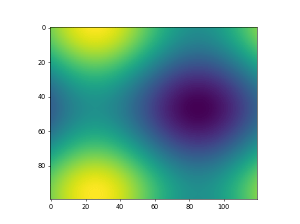Animated image using a precomputed list of images¶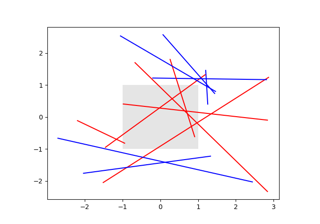Changing colors of lines intersecting a box¶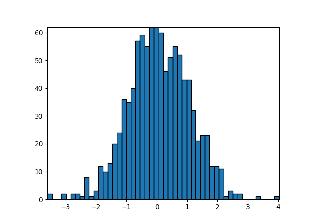Building histograms using Rectangles and PolyCollections¶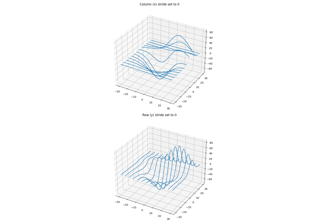3D wireframe plots in one direction¶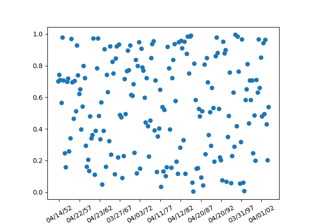Placing date ticks using recurrence rules¶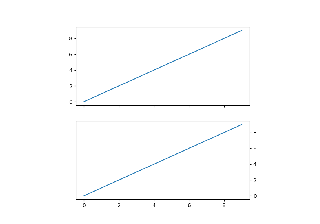Set default y-axis tick labels on the right¶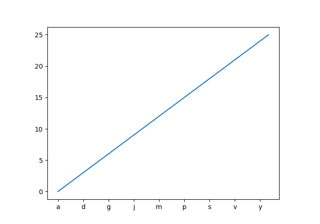Setting tick labels from a list of values¶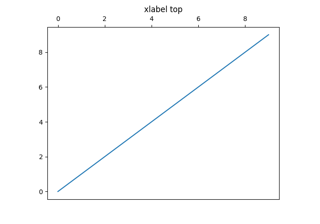Set default x-axis tick labels on the top¶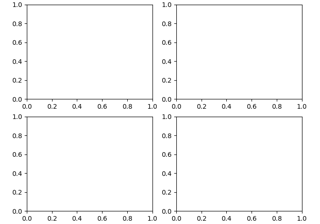Customizing Figure Layouts Using GridSpec and Other Functions¶